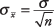## Central Limit Theorem

If the population of all subscribers to the magazine were normal, you would expect its sampling distribution of means to be normal as well. But what if the population were non‐normal? The central limit theorem states that even if a population distribution is strongly non‐normal, its sampling distribution of means will be approximately normal for large sample sizes (over 30). The central limit theorem makes it possible to use probabilities associated with the normal curve to answer questions about the means of sufficiently large samples.

According to the central limit theorem, the mean of a sampling distribution of means is an unbiased estimator of the population mean.Similarly, the standard deviation of a sampling distribution of means isNote that the larger the sample, the less variable the sample mean. The mean of many observations is less variable than the mean of few. The standard deviation of a sampling distribution of means is often called the standard error of the mean. Every statistic has a standard error, which is a measure of the statistic's random variability.

##### Example 1
If the population mean of number of fish caught per trip to a particular fishing hole is 3.2 and the population standard deviation is 1.8, what are the mean and standard deviation of the sampling distribution for samples of size 40 trips?